# Gallery: Introductory examples

## 1) Plot two columns from a file

In this example we show how to quickly plot two columns from a file, change the properties of the resulting curve and plot, and create a postscript version of the figure.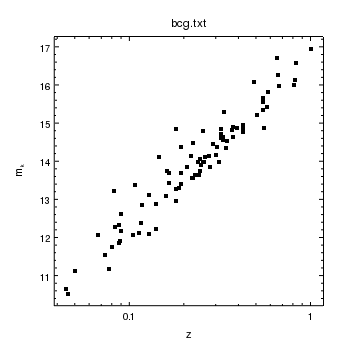Version: Postscript; PDF
make_figure("bcg.txt[cols z,mk]",["line.style","noline"])
set_curve(["symbol.style","square","symbol.size",2])

log_scale(X_AXIS)

set_plot_ylabel("m_k")

print_window("plot")


The make_figure routine creates a plot of the two columns - selected by the Data Model filter - and labels the axes and title. The line.style setting is set to noline to stop lines being drawn connecting the points.

The set_curve routine modifies the existing curve; in this case it changes the symbol to a square of size 2. There are 9 symbol styles to chose from.

The log_scale routine is used to change the scaling of the X axis. The make_figure call uses the column names as axis labels. Here we change the Y-axis label to m_k, where the underscore is used to indicate a subscript. Labels, including axis labels and plot titles, support LaTeX-like syntax for formatting the text.

Finally the print_window routine is used to create a postscript version of the visualization called plot.ps. The output formats are: postscript, encapsulated postscript, PDF, PNG, and jpeg. When used in an interactive Python shell, such as ChIPS or Sherpa, a winow can be used to set the options by saying:

chips> print_window(["printdialog", True])

or by using the Print options from the ChIPS GUI.

#### The ChIPS GUI

CIAO 4.4 added the ChIPS GUI which lets you change a visualization from a GUI. Once a ChIPS window exists, you can show the GUI either by entering the command

chips> show_gui()

at the command line or by pressing the right-mouse button in the ChIPS window to bring up a menu and selecting the "Show GUI" option.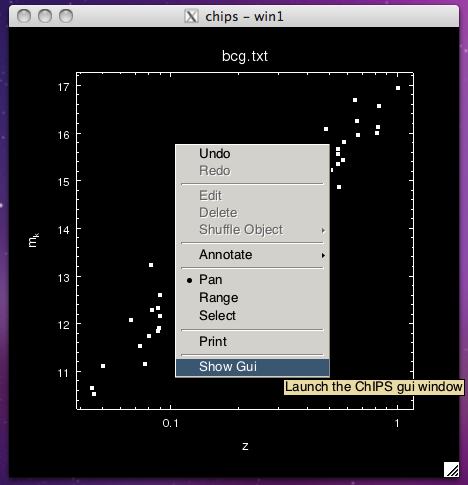The GUI looks like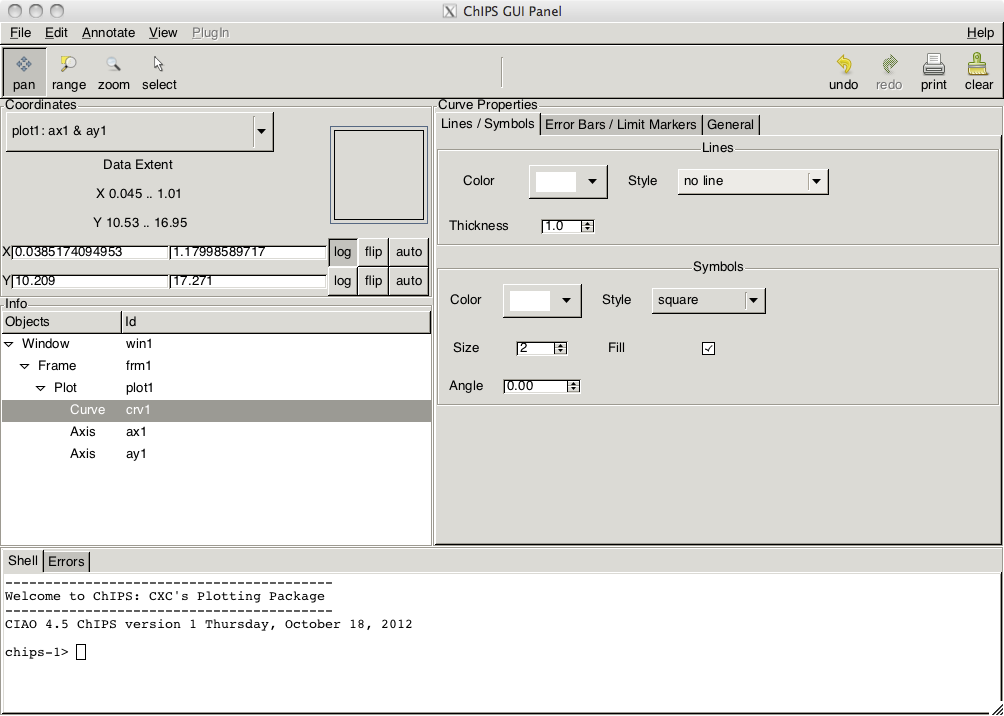When in 'Select' mode, objects in the ChIPS window can be selected, which causes a set of handles to be displayed around the object. These handles can be used to move, resize, or rotate the object, depending on the object type. Once selected, the right-mouse button menu can be used to bring up a window with just that objects properties, as shown below: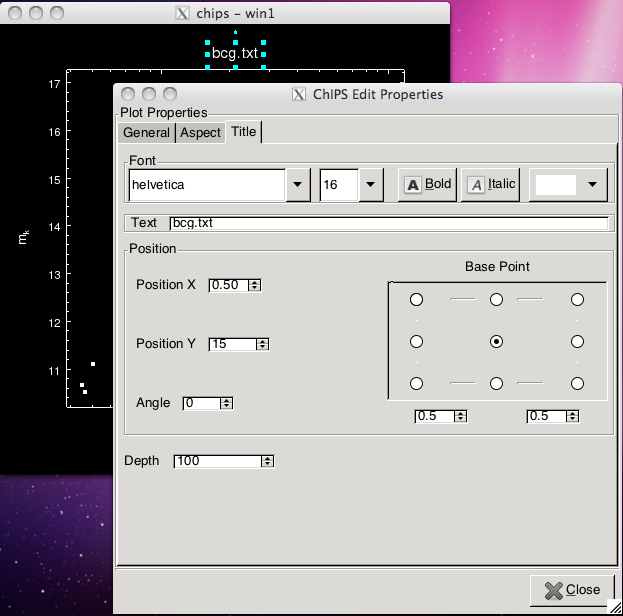## 2) A radial profile drawn as a histogram

In this example we show how to draw a histogram, change the X-axis range and the plot style, and create both postscript and PNG versions of the plot.Version: Postscript; PDF
make_figure("rprof.fits[cols r,sur_bri]","histogram")

log_scale()
limits(X_AXIS,1,AUTO)

set_plot(["style","open"])

print_window("rprof")
print_window("rprof.png")


The make_figure routine can be used to draw a histogram, by adding the optional second argument "histogram". If given two columns then it uses them as x and y values. In this case the first column, r, is a two-dimensinal array, so it is used to indicate the low and high values of each bin. The file was created by dmextract, and the columns are:

unix% dmlist "rprof.fits[cols r,sur_bri]" cols

--------------------------------------------------------------------------------
Columns for Table Block HISTOGRAM
--------------------------------------------------------------------------------

ColNo  Name                 Unit        Type             Range
1   R                 pixel        Real8(2)       -Inf:+Inf            Radius
2   SUR_BRI              count/pixel**2 Real8          -Inf:+Inf            Net Counts per square pixel


Since the data covers a large range, we change the scale from linear to logarithmic for both axes using log_scale, and then use limits to set the minimum X value to 1 (the first bin starts at r=0).

The plot style is changed to open, which hides the top and right axes. See the other examples, particlularly the Axis styles and grids section for more examples of plot styles.

We create postscript and PNG versions of the plot - called rprof.ps and rprof.png respectively - using print_window.

## 3) Contour an image using the WCS axes

In this example we show how to contour an image, adjust the axes to use sexagesimal notation for the labels, and create a postscript version that is US-letter sized.

The next example shows how you can display the data as an image.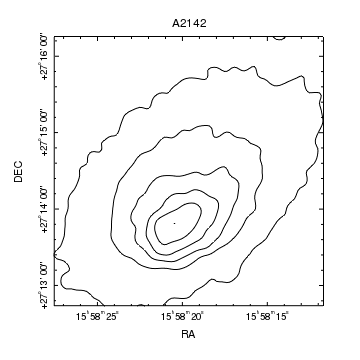Version: Postscript; PDF
make_figure("a2142_smoothed.fits")

set_xaxis(["tickformat","ra"])
set_yaxis(["tickformat","dec"])

set_yaxis(["ticklabel.angle",90])
set_yaxis(["ticklabel.halign",0.5,"ticklabel.offset",15])

print_window("contour",["pagesize","letter","fittopage",True])


If sent an image then make_figure will contour it. As we do not supply any levels, ChIPS uses equally-spaced values that cover the image range. The axis limits are chosen to enclose the outer contour values, not the input image.

Since the image contains a tangent-plane world coordinate system - as shown below by the ahelp opt=cols call - the axes are drawn using this coordinate system. The sky coordinate contour example shows how to use a different coordinate system for the axes.

unix% dmlist a2142_smoothed.fits cols

--------------------------------------------------------------------------------
Columns for Image Block CONVOLVE
--------------------------------------------------------------------------------

ColNo  Name                 Unit        Type             Range
1   CONVOLVE[366,366]                 Real4(366x366) -Inf:+Inf

--------------------------------------------------------------------------------
Physical Axis Transforms for Image Block CONVOLVE
--------------------------------------------------------------------------------

Group# Axis#
1   1,2    sky(x) = (+3109.50) +(+4.0)* ((#1)-(+0.50))
(y)   (+2939.50)  (+4.0)  ((#2) (+0.50))

--------------------------------------------------------------------------------
World Coordinate Axis Transforms for Image Block CONVOLVE
--------------------------------------------------------------------------------

Group# Axis#
1   1,2    EQPOS(RA ) = (+239.5378)[deg] +TAN[(-0.000136667)* (sky(x)-(+4096.50))]
(DEC)   (+27.2755 )           (+0.000136667)  (   (y) (+4096.50))


We change the tick labels to use sexagesimal notation and rotate the tick labels on the Y axis to be parallel to the axis. In the example we use multiple calls to set_yaxis but we could have used the following to change all the attributes in a single call:

ay = ChipsAxis()
ay.tickformat = "dec"
ay.ticklabel.angle = 90
ay.ticklabel.halign = 0.5
ay.ticklabel.offset = 15
set_yaxis(ay)


The print_window routine is used to create a US-letter sized postscript plot called contour.ps, and the figure is expanded so that it fits this page size. Note that the output size can only be changed for the postscript and PDF formats; it does not change the size of images created in PNG or JPEG formats.

#### WCS projection support

In CIAO 4.10, WCS support in ChIPS is only available for tangent-plane projections (e.g. images with RA--TAN and DEC-TAN images). Other projections - such as the SIN projection - will be displayed in physical, or logical coordinates. For the gallery plots, SIN projection images (such as provided by NVSS) have been converted to TAN projection - using a combination of the remap program from WCSTools and the Montage package.

## 4) Display an image with a color bar

In this example we show how to display an image of the same data used in the previous example. We change the colormap of the image (the default is to display a grayscale image), and add a vertical color bar to the plot.Version: Postscript; PDF
make_figure("a2142_smoothed.fits","image")
set_image(["colormap","hsv"])

# Add a colorbar to the plot area
set_colorbar(["*.color","white"])

# Adjust the axis formatting
set_xaxis(["tickformat","ra1"])

set_yaxis(["tickformat","dec1","ticklabel.angle",90])
set_yaxis(["ticklabel.halign",0.5,"ticklabel.offset",15])

# Change the axes and borders to be visible in hardcopy plots
axis = ChipsAxis()
axis.color = "white"
axis.minortick.color = "white"
axis.majortick.color = "white"
axis.ticklabel.size = 14
axis.depth = 150
set_axis("all",axis)

# Create postscript and PNG versions
print_window("image",["pagesize","letter","fittopage",True])
print_window("image.png")


The "image" option of make_figure will display an image of the data (without the option the make_figure call would create a contour plot of the data). The default color map is grayscale, which we change to "hsv" using the set_image call (see the discussion of color maps for more information).

The add_colorbar call adds a color bar to the frame; here we place a vertical bar (the default is horizontal) it to the right-hand side of the plot (x=0.8), centered at y=0.35 and with a height of 0.4 (the length attribute). The positions and length values are given in plot-normalized coordinates. The command

add_colorbar(1.05, 0.5, ["orientation", "vertical"])


would place the color bar just to the right of the plot, and have the same height as the plot.

Since the color bar is placed over the image, we change the color attributes of the color bar to white, rather than default, so that hardcopy outputs - e.g. anything created by print_window - will have readable labels. Without this call the output would likely have black labels (the default value means that the foreground.file preference setting would be used, and this defaults to black) on a black background!

The axes are changed from decimal to sexagesimal notation by setting the tickformat attribute to one of the ra/dec formats (see the Tick Format section of the ChIPS Dictionary for more information on the support formats).

To ensure that the axes and borders are visible, we increase their depth to 150 (the default is 100), the size of the ticklabels and change the color of the non-text axis elements to white.

## 5) Two curves in a plot

In this example we show how to overlay multiple curves within a single plot and how to use the ChipsCurve object to change - or set - the appearance of a curve.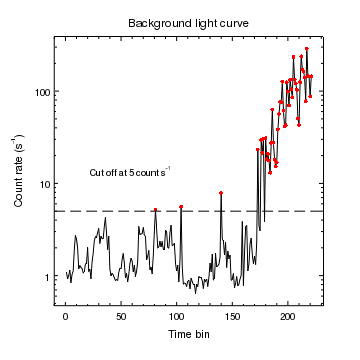Version: Postscript; PDF
make_figure("flare.fits[cols time_bin,count_rate]",["symbol.style","none"])

crv = ChipsCurve()
crv.line.style = "noline"
crv.symbol.style = "circle"
crv.symbol.size = 2
crv.symbol.color = "red"
set_curve(crv)

log_scale(Y_AXIS)

set_plot_xlabel("Time bin")
set_plot_ylabel("Count rate (s^{-1})")
set_plot_title("Background light curve")

add_label(22,12,"Cut off at 5 count s^{-1}")


In this example we use make_figure to plot the initial data - the solid line - and then add to the plot using add_curve. This is because make_figure always erases any existing plots, whereas add_curve will add the curve to an existing plot.

In this example we use the ChipsCurve object to change a number of the second curve's attributes in one call to set_curve. We could have also used the crv variable in the add_curve call by saying

add_curve("flare.fits[count_rate>5][cols time_bin,count_rate]", crv)

The add_hline() routine is used to add a horizontal line - in this case drawn with long dashes - that always extends across the whole plot, no matter what range is selected for the X axis.

## 6) Multiple histograms within a plot

In this example we show how to draw multiple histograms within a single plot and how to add annotations (lines and labels).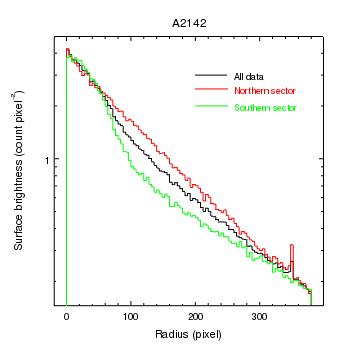Version: Postscript; PDF
make_figure("rprof.fits[cols r,sur_bri]","histogram")

log_scale(Y_AXIS)

set_plot_ylabel("Surface brightness (count pixel^{-2})")



Three datasets are plotted in this figure. The first is created using make_figure while the remaining are created using add_histogram, since make_figure() will erase any existing plots when called.

The axis labels are changed from the values created by make_figure(), and include LaTeX-like commands for formatting the text. Finally labels and lines are used to annotate the plot. The valign attribute of the labels is set to 0.5 so that they are centered vertically on the lines. Regions can also be used to help annotate plots, as shown in the Two sets of contours example.

Although the annotations are placed explicitly in this example, they can also be added directly from the GUI, either from the RMB menu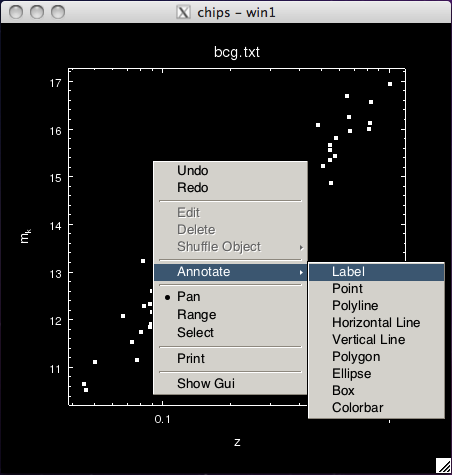or from the main GUI window, using the Annotate menu: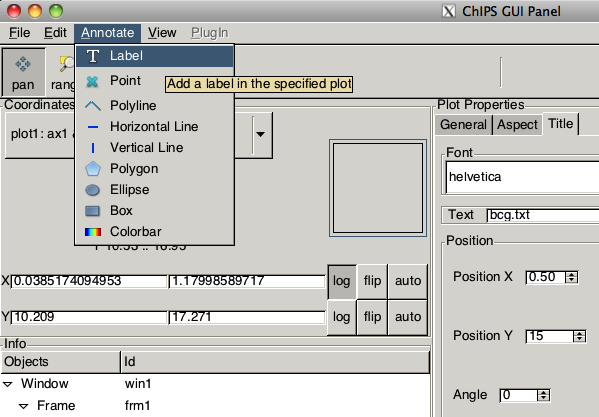## 7) Reading in data from FITS and ASCII files

In this example we plot up data points read from a FITS file and an ASCII file that uses tab-separated values (e.g. as created by the catalog option in ds9), as well as displaying contours that were also created by ds9. Annotations - namely labels and symbols - are added to the top of the plot in lieu of a title.

For data input we plot the RA and Dec positions of sources found from Chandra data - using the RA_SRC and DEC_SRC virtual columns created by wavdetect

unix% dmlist src.315.fits"[cols pos]" cols

--------------------------------------------------------------------------------
Columns for Table Block SRCLIST
--------------------------------------------------------------------------------

ColNo  Name                 Unit        Type             Range
1   POS(X,Y)             pixel        Real8          3536.50:     4825.50 Physical coordinates

--------------------------------------------------------------------------------
World Coord Transforms for Columns in Table Block SRCLIST
--------------------------------------------------------------------------------

ColNo    Name
1:    EQSRC(RA_SRC ) = (+180.4861)[deg] +TAN[(-0.000136667)* (POS(X)-(+4096.50))]
(DEC_SRC)   (-18.8405 )           (+0.000136667)  (   (Y) (+4096.50))



and 2MASS positions from the RAJ2000 and DEJ2000 columns in the tab-separated ASCII file created by ds9:

chips> dmlist 2mass.315.tsv"[opt kernel=text/tsv]" cols

--------------------------------------------------------------------------------
Columns for Table Block 2mass.315.tsv
--------------------------------------------------------------------------------

ColNo  Name                 Unit        Type             Range
1   _RAJ2000                          Real8          -Inf:+Inf
2   _DEJ2000                          Real8          -Inf:+Inf
3   RAJ2000                           Real8          -Inf:+Inf
4   DEJ2000                           Real8          -Inf:+Inf
5   2MASS                             Int4           -
6   Jmag                              Real8          -Inf:+Inf
7   e_Jmag                            Real8          -Inf:+Inf
8   Hmag                              Real8          -Inf:+Inf
9   e_Hmag                            Real8          -Inf:+Inf
10   Kmag                              Real8          -Inf:+Inf
11   e_Kmag                            Real8          -Inf:+Inf
12   Qflg                              String
13   Rflg                              Int4           -
14   Bflg                              Int4           -
15   Cflg                              String
16   Xflg                              Int4           -
17   Aflg                              Int4           -Version: Postscript; PDF
add_curve("src.315.fits[cols ra_src,dec_src]")

# Reverse the X axis so that RA is decreasing to the right
reverse_axes(X_AXIS)

# Change the curve properties
set_curve("all",["line.style","noline"])
set_curve("crv1",["symbol.style","circle"])
crv = ChipsCurve()
crv.symbol.style = "square"
crv.symbol.fill = False
crv.symbol.color = "orange"
set_curve("crv2",crv)

from chips_contrib.utils import add_ds9_contours

# zoom in to better see the sources in NGC4038/9
zoom(2)

# Label the plot where the title would be
set_label("all",["size",16])

# adjust the axis tick labels to use sexagesimal notation
set_xaxis(["tickformat","%ra1"])
set_yaxis(["tickformat","%dec1"])


The add_curve command is used to plot up the RA versus Dec positions from the two files, using DataModel syntax to select the columns to plot and select the text format for reading the ASCII file.

Since no projection information is given, the axes are created as "normal" 2D axes; that is the data is assumed to lie on the cartesian plane, and there is no recognition that Right Ascension values should be displayed in descending order. We therefore use the reverse_axes routine to ensure that the X axis is displayed as expected. Note that it is possible to create a pair of axes that represent a tangent-plane projection, as shown in several of the image examples in this section.

Once both data sets have been displayed, we use the set_curve command to hide the lines between the points (for both curves), and then change the symbol style, color, and fill for the two curves (the Chandra data set is crv1 and 2MASS is crv2). At this point the info output is:

chips> info()

Window [win1]
Frame [frm1]
Plot [plot1]   (0.15,0.15)  .. (0.90,0.90)
Border bottom [bx1]  top [bx2]  left [by1]  right [by2]
Curve [crv1]
X Axis [ax1]
Y Axis [ay1]
Curve [crv2]



The add_ds9_contours routine from the chips_contrib.utils module is used to display the contour file created by ds9, which looks like

chips> !head -5 antennae.nvss.con
1.80482241e+02 -1.88954887e+01
1.80484947e+02 -1.88947398e+01
1.80489331e+02 -1.88913216e+01
1.80489351e+02 -1.88913034e+01
1.80493324e+02 -1.88871546e+01


into a curve, which we display using the forest color (a dark green). Note that the contour file is assumed to contain RA and Dec values in fk5 format.

The zoom command is one of many routines that can be used to change the limits of the axes.

Instead of a plot title, we use a combination of add_point and add_label calls to add annotations to the plot. These are all placed in plot-normalized coordinates, so that they span the width of the plot and appear just above it (since y=1.05). The labels are positioned so that they are vertically aligned with the points, by setting valign=0.5, and the 'NVSS contours' label is right-aligned to the plot edge by setting halign=1 (the default for labels is to be left-aligned, i.e. halign=0). At this point the info output is:

chips> info()

Window [win1]
Frame [frm1]
Plot [plot1]   (0.15,0.15)  .. (0.90,0.90)
Border bottom [bx1]  top [bx2]  left [by1]  right [by2]
Curve [crv1]
X Axis [ax1]
Y Axis [ay1]
Curve [crv2]
Curve [ds9con3]
Point [pnt1]
Label [lbl1]
Point [pnt2]
Label [lbl2]
Label [lbl3]


Finally we change the axes to use sexagessimal notation, taking advantage of the variety of available formats.

## 8) Use the sky coordinate system to contour an image

In this example we show how to contour an image - selecting the SKY coordinate system rather than the default celestial one - and how to add a filled region, ensuring that it does not obscure the contours (which is only necessary if the visualization is going to be saved to PS or EPS format).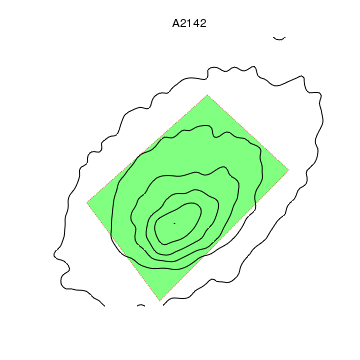Version: Postscript; PDF
make_figure("a2142_smoothed.fits",["wcs","sky"])

# Add a region
xr = [3840,3970,3764,3647]
yr = [3970,3850,3641,3798]

# Hide the axes and the borders
hide_axis()
set_plot(["style","open"])


By setting the wcs parameter to "sky", the contour is drawn using the SKY coordinate system (the value "physical" produces the same plot).

A region is added to the plot by specifying the four corners; for general polygons the add_region routine takes two one-dimensional arrays containing the x- and y- coordinates of the vertexes respectively. Since the polygon is convex we can fill it; we also change the edge color and style to highlight the region. The depth attribute is set to 50 for the region so that it is drawn behind the contours, which are drawn at the default depth of 100. The plot within a plot example shows another way of changing the order objects are drawn, and the Depth Control concept document provides more information on this topic.

Since the region fill is, by default, partially transparent (the region.opacity is set to 0.5) then the contours will still be visible on screen even if the depth setting is not changed. This is also true for the PDF and bitmap formats created by the print_window command, but it does not hold for postscript and EPS versions, which only create fully-opaque fill colors.

To finish with, the axes and plot edges are hidden. If the plot style had been set to boxed rather than open a solid rectangle, with no tick marks, would have surrounded the plot (e.g. see Example 3 of the Axis styles and grids section).

#### Opacity and Postscript output

Note that the postscript output created by print_window does not support opaque region or histogram fills; instead the opacity is taken to be 1. The relative depth of the objects can be changed - by altering the depth attribute or using the various "shuffle commands" (shuffle, shuffle_back, shuffle_front, shuffle_backward, shuffle_forward, and the set of shuffle_<object> routines) so that overlapping objects are not completely obscured if desired.

## 9) Display an image and overlay contours

In this example we show how to display an image and then overlay contours on it. For the image we use a Chandra observation of the galaxy cluster, A2142, as used in the previous image example, but this time zoom in on a region which also shows radio emission. We overplot contours from the FIRST (brown) and NVSS (orange, dashed) catalogs.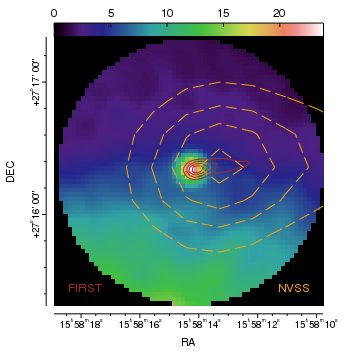Version: Postscript; PDF
make_figure("a2142_smoothed.fits[sky=circle(15:58:14.3,27:16:19,1')]","image")
set_image(["colormap","hsv"])

# FIRST contours

# NVSS contours

# Add a colorbar
set_plot_title("")

# Annotate the plot
set_label("all",["size",16])

# Change the axes
set_xaxis(["tickformat","ra"])
set_yaxis(["tickformat","dec"])

set_yaxis(["ticklabel.angle",90])
set_yaxis(["ticklabel.halign",0.5,"ticklabel.offset",15])

# Move the axes away from the image and hide the plot border
move_axis("ax1",0,-0.03)
move_axis("ay1",-0.03,0)
set_plot(["style","open"])

# Change the axes so that the tick marks point away from the plot
set_axis(["tickstyle","outside"])

print_window("combined.pdf",["pagesize","letter","fittopage",True])
print_window("combined.png")


The make_figure call is used to display the Chandra image: we apply a Data Model spatial filter to restrict the display to the region of interest. Two contours are then overlaid on this image using the add_contour command. Since the image and contours are placed using the WCS data in the files, the images do not need to have the same pixel scale.

The title is removed using the set_plot_title command and a color bar for the image is added above the plot using the add_colorbar command. The color bar coordinates (here 0.5,1.0) refer to the alignment point, determined by the halign and valign attributes. As we want the bar to appear directly above the plot we set valign to 0.

Labels are added to the bottom of the plot, placed using the plot-normalized coordinate system, and colored to match the contours. The "NVSS" label is positioned so that its right edge is at x=0.95 (i.e it is right-aligned), by setting its halign attribute to 1.

The axis labels are changed to use sexagesimal notation, Y axis labels rotated so that they are parallel to the axis, the tickmarks made to appear outside the plot rather than inside, and then the axes are moved slighly away from the plot (with the move_axis call).

#### WCS projection support

In CIAO 4.10, WCS support in ChIPS is only available for tangent-plane projections (e.g. images with RA--TAN and DEC-TAN images). Other projections - such as the SIN projection - will be displayed in physical, or logical coordinates. For the gallery plots, SIN projection images (such as provided by NVSS) have been converted to TAN projection - using a combination of the remap program from WCSTools and the Montage package.

## 10) Placing one plot within another plot

In this example we show how to create multiple plots by placing one plot within another, find out the data range covered by a curve, switch between plots, and create a filled region.

Several other examples indicate the use of multiple plots in a page, for instance the COSMOS observations and WCS Axis grid visualizations.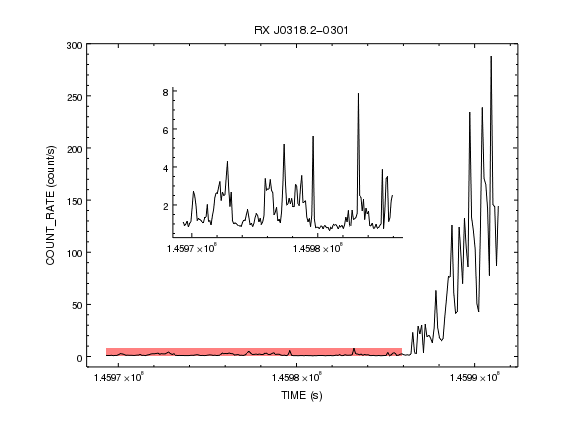Version: Postscript; PDF
set_preference("curve.symbol.style","none")
make_figure("flare.fits[cols time,count_rate]")

set_xaxis(["majortick.interval",1e4,"tickformat","%Z"])

set_xaxis(["majortick.interval",1e4,"tickformat","%Z"])

xr = get_curve_xrange()
yr = get_curve_yrange()

current_plot("plot1")

x = [xr, xr, xr, xr]
y = [yr, yr, yr, yr]

shuffle_region(chips_back)
limits(Y_AXIS,-10,300)


Since we know we do not want symbols to be drawn for curves, we set the curve.symbol.style preference to none here, rather than changing the symbol.style attribute for every curve we create. We also use the add_window call to create a rectangular window to draw in (the default is to create a square window, as can be seen in the previous examples).

Since the time values are large, we change the tick mode of the X axis so that a major tick mark is drawn every 10^4 seconds, and the tickformat so that exponential notation is used instead of text like 1.4597e+08.Note

The dmextract tool also creates a DT column when opt=ltc1 which gives the time in seconds from the start of the observation; this column could have been used here instead of TIME.

To highlight the behavior in the low-count region a second plot is added within the first one. We use the add_plot routine to place the plot roughly in the center of the existing plot, and change the plot.style attribute to open to hide the display of the top and right axes. The add_curve call adds the curve to this new plot rather than the original plot, since it is the one that is current. The file name includes a Data Model filter on the time column to restrict the data being plotted.

We add a region to the first plot showing the range covered by the second plot. This is done by first finding out the data range of the second curve using the get_curve_xrange and get_curve_yrange calls. The currency is then changed back to the first plot using current_plot, and then the region is drawn using the coordinates obtained from the second plot. The region is moved so that it is drawn behind the curve by the shuffle_region call. The contour using sky coordinates example shows an alternative way of changing the order objects are drawn.

An alternative way to create this plot would have been to read the data into Python - as in the strip chart example - and plot the array values, instead of reading the data from a file both times. In this case the calls would have looked something like:

flare = read_table("flare.fits")
t = copy_colvals(flare, "TIME")
crate = copy_colvals(flare, "COUNT_RATE")


and

idx, = np.where(t < 1.45986e8)


### Controlling the size of the plot output

The print_window command allows you to control the output size of your visualiztion when using the postscript and PDF output formats (the bitmap formats are fixed to match the window size). Use the print GUI, e.g.

chips> print_window(["printdialog", True])


or using the pagesize and fittopage attributes as shown in the contour example plot above.

## 11) Plotting related values in a strip chart

In this example we show how to create multiple plots, read in data from a file, manipulate it, and place labels outside the plots.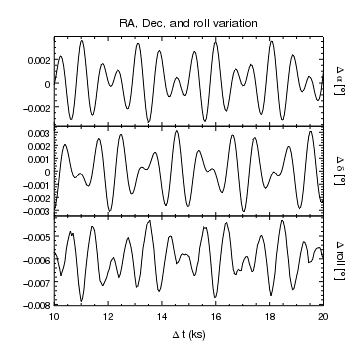Version: Postscript; PDF
tbl = read_file("asol1.fits")
t = copy_colvals(tbl,"time")
ra = copy_colvals(tbl,"ra")
dec = copy_colvals(tbl,"dec")
roll = copy_colvals(tbl,"roll")

dt = (t - t)/1e3
dra = ra - get_keyval(tbl,"RA_NOM")
ddec = dec - get_keyval(tbl,"DEC_NOM")
droll = roll - get_keyval(tbl,"ROLL_NOM")

# We now use linear interpolation to reduce the number of
# points to plot.
x = np.linspace(10, 20, 201)
yra = np.interp(x, dt, dra)
ydec = np.interp(x, dt, ddec)
yroll = np.interp(x, dt, droll)

pref = ChipsPreferences()
pref.curve.symbol.style = "none"
pref.label.size = 14
pref.label.halign = 0.5
pref.label.angle = 270
set_preferences(pref)

strip_chart(3)

set_plot_title("RA, Dec, and roll variation")

current_plot("plot2")

current_plot("plot3")

set_plot_xlabel(r"\Delta t (ks)")

# Ensure the whole width of the plot is used to display data


Since the time values will be used in all three plots we read the file in once - using the read_file routine - and plot the arrays each time. The copy_colvals routine gets the array data for the given column from the return value of read_file (here the variable tbl). Having read in the arrays means that we can perform some simple scaling so as to simplify the range of each plots; the time value is converted to an offset from the first value and changed from seconds to kiloseconds, and the other values are turned into offsets from the *_NOM header keyword values.

The aspect solution is sampled roughly every quarter of a second, which means that it contains a large number of points for the chosen display range of 10 to 20 kiloseconds. We therefore use linear interpolation to re-bin the data to a sample rate of 50 seconds using the np.interp routine from NumPy.

The ChipsPreference object and set_preferences call are used to set up several attribute preferences, such as no symbols for curves.

The drawing begins with the strip_chart call, which creates three vertical plots. The top plot - called "plot1" - is the current plot, so is where the x,yra values will be drawn. A label indicating the Y axis is added to the right-hand side of the plot using add_label. Since we want the label to appear outside the plot we use the plot normalized coordinate system to place the label to the right of the plot (x=1.05), and mid-way up it (y=0.5). The earlier preference settings ensure that the label is centered on this position (label.halign=0.5) and rotated (label.angle=270). Since the label text contains \ characters, it is preceeded by the r symbol to ensure it is interpreted correctly.

The remaining commands move to the second and third plots (plot2 and plot3 respectively), using the current_plot routine, and add data - a curve and a label - to each of them.

## 12) Using a filled region (solid) to show the error bounds

In this example we use a region to represent the error bounds of a set of points. The interior of the region is drawn with a solid-fill pattern; is is also possible to use a range of patterns to fill a region instead.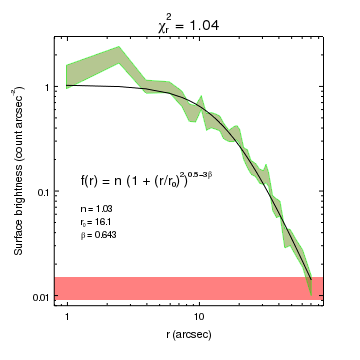Version: Postscript; PDF
rprof = read_file("profile.fits")
r = copy_colvals(rprof,"R")
y = copy_colvals(rprof,"CEL_BRI")
dy = copy_colvals(rprof,"CEL_BRI_ERR")

x = 0.492 * 0.5*(r[:,0] + r[:,1])
ym = 1.03 * (1 + (x/16.1)**2)**-1.43

xr = np.append(x, x[::-1])
yr = np.append(y+dy, (y-dy)[::-1])

log_scale()
limits(Y_AXIS,0.008,3)

bx = [0.1, 1000, 1000, 0.1]
by = [0.009, 0.009, 0.015, 0.015]

set_plot_xlabel("r (arcsec)")
set_plot_ylabel("Surface brightness (count arcsec^{-2})")
set_plot_title(r"\chi^2_r = 1.04")
set_plot(["title.size",20])

add_label(0.1,0.45,r"f(r) = n (1 + (r/r_0)^2)^{0.5-3\beta}",["coordsys",PLOT_NORM,"size",18])


The input file used here (profile.fits) contains a radial profile created by dmextract. The columns we are interested in are R - which gives the minimum and maximum radius of each annulus in pixels - and the surface brightness columns CEL_BRI and CEL_BRI_ERR, which are in counts per square arcsecond. Note that the last two are stored as virtual columns, as shown in the dmlist output below:

unix% dmlist "profile.fits[cols r,sur_bri,sur_bri_err]" cols

--------------------------------------------------------------------------------
Columns for Table Block HISTOGRAM
--------------------------------------------------------------------------------

ColNo  Name             Unit           Type          Range
1   R             pixel          Real8(2)   -Inf:+Inf        Radius
2   SUR_BRI          count/pixel**2 Real8      -Inf:+Inf        Net Counts per square pixel
3   SUR_BRI_ERR      count/pixel**2 Real8      -Inf:+Inf        Error on net counts per square pixel

--------------------------------------------------------------------------------
World Coord Transforms for Columns in Table Block HISTOGRAM
--------------------------------------------------------------------------------

ColNo    Name
2:    CEL_BRI          = +0 [count/arcsec**2] +4.1311 * (SUR_BRI  -0)
3:    CEL_BRI_ERR      = +0 [count/arcsec**2] +4.1311 * (SUR_BRI_ERR  -0)


However, we do not need to treat them in any different way to access the values (prior to CIAO 4.4 the column names needed to be included in the read_file call if the column was virtual).

The bin center is calculated from the R values and converted to arcseconds, using the default ACIS pixel scale of 0.492 arcseconds per pixel. The model fit - a one-dimensional beta profile - is calculated using the bin center values and drawn as a curve.

To draw the data values we create a polygon by appending the (x,y-dy) values - in reverse order - onto the (x,y+dy) values to form the xr and yr arrays, and then use these to create a filled region. The depth attribute is set to 90 so that the region is drawn behind the other plot elements (which are drawn at the default depth of 100). This ensures that the curve is visible postscript hardcopy output - created by print_window. Changing the depth is not necessary if only PDF or bitmap (PNG or JPEG) formats are required.

The region was added to the plot after the curve to make sure that there was a data coordinate system to use. Unlike curves, histograms, and contours, creating a region will not create a pair of axes if one does not already exist.

Labels have been added to the plot to indicate the form, and numerical values, of the model line. Rather than use the data coordinate system - i.e. the values on the axes - to position the labels, the PLOT_NORM coordinate system is used. This means that a coordinate of 0.1,0.25 refers to a point ten percent in from the left X axis and a quarter of the way up the plot.

#### Opacity and Postscript output

Note that the postscript output created by print_window does not support opaque region or histogram fills; instead the opacity is taken to be 1. The relative depth of the objects can be changed - by altering the depth attribute or using the various "shuffle commands" (shuffle, shuffle_back, shuffle_front, shuffle_backward, shuffle_forward, and the set of shuffle_<object> routines) so that overlapping objects are not completely obscured if desired.

## 13) Filling a region with a pattern to show the error bounds

In this example we replicate the plot from the Using a a filled region to show the error bounds example, but this time use a pattern to full the region.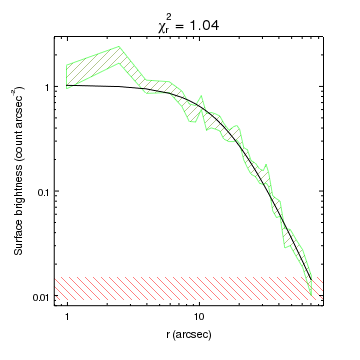Version: Postscript; PDF
rprof = read_file("profile.fits")
r = copy_colvals(rprof,"R")
y = copy_colvals(rprof,"CEL_BRI")
dy = copy_colvals(rprof,"CEL_BRI_ERR")

x = 0.492 * 0.5*(r[:,0] + r[:,1])
ym = 1.03 * (1 + (x/16.1)**2)**-1.43

xr = np.append(x, x[::-1])
yr = np.append(y+dy, (y-dy)[::-1])

log_scale()
limits(Y_AXIS,0.008,3)

bx = [0.1, 1000, 1000, 0.1]
by = [0.009, 0.009, 0.015, 0.015]

set_plot_xlabel("r (arcsec)")
set_plot_ylabel("Surface brightness (count arcsec^{-2})")
set_plot_title(r"\chi^2_r = 1.04")
set_plot(["title.size",20])


The fill.style attribute of regions is used to determine how the region is filled. Here we use the values "updiagonal" and "downdiagonal" to fill the two regions with diagonal lines.

## 14) Display a three-color image

This example creates a three-color image - also known as a true-color image - in a similar manner to the dmimg2jpg tool.

For the three images we use the soft (0.5 to 1.5 keV), medium (1.5 to 3 keV), and hard (3 to 8 keV) bands of a Chandra observation of Cassiopeia A. We use the event file from the archive (renamed to obsid4638.fits), and apply Data Model filters and binning to extract the required data. The choice of bands and binning factor are for display purposes only, and were not chosen for any scientific reason.Version: Postscript; PDF
# Load in the scale_image_crate routine
from crates_contrib.utils import *

# Create three images in soft, medium, and hard bands,
# scaling the data before display
fname = "obsid4638.fits[energy={0}:{1}][bin x=3589.5:5063.5:2,y=3157.5:4634.5:2]"

scale_image_crate(r, "sqrt")
scale_image_crate(g, "sqrt")
scale_image_crate(b, "sqrt")

# Create the three-color image

# Annotate the visualization
set_plot_title("Cassiopeia A, ObsID 4638")
set_plot(["title.size",18])

set_xaxis(["tickformat","ra"])
set_yaxis(["tickformat","dec"])

# Change the axes and borders of the plot
axis = ChipsAxis()
axis.majortick.style = "outside"
axis.minortick.style = "outside"
axis.ticklabel.size = 18
axis.depth = 150
set_axis("all",axis)

# Calculate a bar with a length of 5 arcminutes
x1 = 351
y1 = 58.75
x2 = x1 - 5.0/(np.cos(y1*np.pi/180) * 60)

xmid = 0.5*(x1+x2)
y2 = y1 - 0.005

print_window("casa.png")


The add_image command can be used to display a single image, as in a previous example, but it can also be used to create a three-color image, as is done here.

Prior to calling add_image(), we first scale the data using the scale_image_crate routine from the crates_contrib.utils module. For this dataset, using the square-root of the pixels produces a good balance between showing low-level features whilst retaining definition in the high signal areas. This step is necessary because the image support in CIAO 4.7 only provides a linear display range, so you need to scale the data before displaying it. The Overlay Chandra data on an optical image example shows how you can manually scale image data for use by add_image().

A scale bar is created in the bottom-left corner of the plot to indicate the image scale. The line and label are set to white so that they remain visible in the hardcopy outputs.

## 15) Re-sizing a plot to match the aspect ratio of an image

Here we show how the plot can be re-sized to match the data - in this case an image - by setting the plot's aspect ratio.

The image being displayed is a subset of a HRC-S observation of Cas A (ObsId 1068), which has been divided by an exposure map, so has pixel values around 10-5:

unix% dmstat casa_hrc.fluxed centroid-
PFLUX_IMAGE[/cm**2 /s]
min:	0 	      @:	( 29208.5 30552.5 )
max:	0.00032340790494 	      @:	( 32888.5 33544.5 )
mean:	3.2201918066e-06
sigma:	1.0328240814e-05
sum:	0.39211953609
good:	121769
null:	0


and is rectangular

unix% dmlist casa_hrc.fluxed blocks

--------------------------------------------------------------------------------
Dataset: casa_hrc.fluxed
--------------------------------------------------------------------------------

Block Name                          Type         Dimensions
--------------------------------------------------------------------------------
Block    1: PFLUX_IMAGE                    Image      Real4(463x263)Version: Postscript; PDF
add_window(9,9,"inches")
set_image(["threshold",[0, 5e-5]])

# Re-size the plot so that it matches the data
set_plot_aspect_ratio("fit")

# Hide the axes and borders
hide_axis()
set_plot(["style","open"])

# Change the frame background so that we can see it
set_frame(["bgcolor","gray"])

# Make the plot fill the window
reposition_plot(0,0,1,1)


We start by creating a square window, 9 inches on a side, and then display the image, adjusting the threshold range to show the background emission.

At this point the plot has the standard limits - covering from (0.15,0.15) to (0.9,0.9) in frame-normalized coordinates - and so does not match the data, which is roughly twice as wide as it is high. The set_plot_aspect_ratio command is used to adjust the plot area so that it matches the data; that is the Y axis is shrunk so that now it only covers the image.

Additional commands are used to hide the axes and plot borders, change the frame background color from default (black on screen, white for the hardcopy outputs) to gray so that it is more obvious where the plot covers, and then re-size the plot so that it fills the screen. Note that even though the plot borders are now (0,0) to (1,1) in frame-normalized coordinates - e.g. as shown in the info output below

chips> info()

Window [win1]
Frame [frm1]
Plot [plot1]   (0.00,0.00)  .. (1.00,1.00)
Border bottom [bx1]  top [bx2]  left [by1]  right [by2]
Image [image1]
X Axis [ax1]
Y Axis [ay1]



the plot area is still restricted to the image (that is, the gray background can still be seen), as the aspect ratio is still set:

chips> get_plot_aspect_ratio()
'463.0:263.0'


Given this aspect ratio, we could have created a rectangular window, better matching the data, such as

add_window(4.6*2, 2.6*2, 'inches')


## 16) Moving an axis, plotting a cumulative distribution, and using Sherpa

In this example we create a histogram of the count-rate column of a file and plot both it, and the cumulative distribution, on the same graph by having two Y axes.

We also load in the Sherpa module so that we can fit a Gaussian model to the count-rate histogram and display it.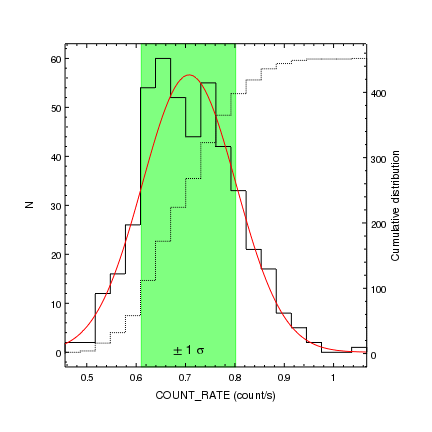Version: Postscript; PDF
# Load in the Sherpa routines
from sherpa.astro.ui import *

# Load in the data (rdata is a CrateData object)
rdata = cr.get_column('count_rate')
rate = rdata.values

# Filter out those points at the start/end where the GTI
# means that the exposure time is 0
frate = rate[rate > 0.0]

# Use the NumPy module to create a histogram of the count rate
(yh, edges) = np.histogram(frate, bins=20)

# Get the bin edges and middle
xlo = edges[:-1]
xhi = edges[1:]
xmid = (xlo + xhi) / 2.0

# Use Sherpa to fit a gaussian to the data
set_source(1, gauss1d.g1)
guess(g1)
fit()

# Evaluate the model on a finer grid than the data
xg = np.linspace(edges.min(), edges.max(), 100)
yg = g1(xg)

# Find the +/- 1-sigma range from the model
ygc = np.cumsum(yg / yg.sum())
xminus = xg[ygc <= (0.5 - 0.683/2)][-1]
xplus  = xg[ygc >= (0.5 + 0.683/2)]

# Now plot up the data
add_curve(xg, yg, ['symbol.style', 'none', 'line.color', 'red'])

# Label the plots
xlbl = "{} ({})".format(rdata.name, rdata.unit)
xlbl = xlbl.replace('_', '\_')
set_plot_xlabel(xlbl)
set_plot_ylabel("N")

# Add an axis to the right-hand side of the plot and
# plot up the cumulative distribution
add_axis(Y_AXIS, 1, 0, 1)
add_histogram(xlo, xhi, np.cumsum(yh), ['line.style', 'dot'])
set_plot(["rightmargin",0.15])
set_plot_ylabel("Cumulative distribution")

# Add a region and label displaying the 1-sigma range
yr = get_plot_yrange()
add_region([xminus, xplus, xplus, xminus], [yr, yr, yr, yr], ['depth', 50])
add_label((xplus+xminus)/2, 0, r'\pm 1 \sigma', ['halign', 0.5, 'size', 18])

# Adjust the X axis so that there is no padding
# - an alternate would be to say
#   limits(X_AXIS, chips_histogram, "hist1")
#


After ensuring that the main Sherpa routines are loaded, we use the read_file routine from crates to load in the data in the file lcurve.fits. The get_column method of the Crate returns a CrateData object representing the COUNT_RATE column; we can use the values field to get a NumPy array and later on we will use the name and unit fields to label the X axis.

Since this light curve starts and ends with several bins for which the exposure time, and hence count rate, are zero, we filter them out to create the frate column, which we pass to the NumPy histogram routine to calculate the histogram values.

We then fit a one-dimensional Gaussian model to the histogram data (using a non-integrated version of the model). Since we have not reduced the verbosity of Sherpa, the following is printed to the screen by the fit command:

Dataset               = 1
Method                = levmar
Statistic             = chi2gehrels
Initial fit statistic = 201.329
Final fit statistic   = 11.035 at function evaluation 33
Data points           = 20
Degrees of freedom    = 17
Probability [Q-value] = 0.854741
Reduced statistic     = 0.649115
Change in statistic   = 190.294
g1.fwhm        0.2236
g1.pos         0.707394
g1.ampl        56.6438


Note that for the purposes of this example we did not change the fit statistic.

After fitting, we can use the g1 model to evaluate the Gaussian on a finer grid than the data. This new set of points (xg,yg) is then used to find the one-sigma range by calculating the cumulative of the normalized distribution and finding out where it reaches 15.85% and 84.15%; these values will be used to highlight the one-sigma region. Note that in this case we could just use the parameters of the g1 model to get this region, but the manual approach can be useful when we do not have an analytic model.

After plotting up the histogram and best-fit Gaussian model, we add labels to the bottom and left axes (since underscores are taken to mean a sub-script, we replace any '_' character with '\_' in the X-axis label to avoid this).

The cumulative distribution is also displayed as a histogram; as it has a different range to the histogram, we first add an extra Y axis to the right-hand side of the plot (the first 1 argument indicates the plot-normalized position of the axis; see add_axis for more inforamtion). The histogram is then added to this axis; since there is only one X axis it uses that which, in this case, is what we want. The right-hand margin of the plot is increased from its default value of 0.05 to ensure there is place for an axis label.

The last part of a plot is a region which covers the one-sigma range of the fit (the height is set to the plot range so that it fills the plot). Its depth is set to 50 so that it does not obscure all the other elements in the postscript output.

#### Opacity and Postscript output

Note that the postscript output created by print_window does not support opaque region or histogram fills; instead the opacity is taken to be 1. The relative depth of the objects can be changed - by altering the depth attribute or using the various "shuffle commands" (shuffle, shuffle_back, shuffle_front, shuffle_backward, shuffle_forward, and the set of shuffle_<object> routines) so that overlapping objects are not completely obscured if desired.